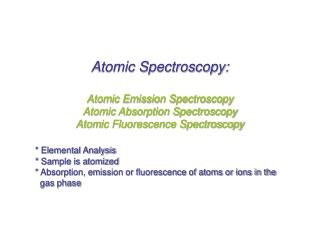DownloadDownload PresentationAtomic Spectroscopy: Atomic Emission Spectroscopy Atomic Absorption Spectroscopy

# Atomic Spectroscopy: Atomic Emission Spectroscopy Atomic Absorption Spectroscopy

Télécharger la présentation## Atomic Spectroscopy: Atomic Emission Spectroscopy Atomic Absorption Spectroscopy

- - - - - - - - - - - - - - - - - - - - - - - - - - - E N D - - - - - - - - - - - - - - - - - - - - - - - - - - -
##### Presentation Transcript

1. Atomic Spectroscopy: Atomic Emission Spectroscopy Atomic Absorption Spectroscopy Atomic Fluorescence Spectroscopy * Elemental Analysis * Sample is atomized * Absorption, emission or fluorescence of atoms or ions in the gas phase

2. Atomic Emission Spectroscopy Ingle and Crouch, Spectrochemical Analysis

3. Electronic Levels for Individual Electrons Ingle and Crouch, Spectrochemical Analysis

4. Electronic Configuration of Atoms Al: 1s2 2s2 2p6 3s2 3p1

5. 1s 2s 2p 3s 3p 3d 4s 4p 4d 4f 5s 5p 5d 5f 6s 6p 6d 6f Electronic Configuration of Atoms Aufbau Order l = 0 s-orbital ml = 0 l = 1 p-orbital ml = 0, ±1 l = 2 d-orbital ml = 0, ±1, ±2

6. Electronic Configuration of Atoms Al: 1s2 2s2 2p6 3s2 3p1

7. Electronic States of Atoms Quantum numbers for electronsQuantum numbers for many-electron atoms l: orbital angular momentum quantum L: orbital angular momentum quantum number number (0,1, … n-1 e.g., for 2 e-: L = l1+l2, l1+l2 -1, l1+l2 -2, …,| l1-l2 | where 0=s, 1=p, 2=d, 3=f) 0 = S, 1 = P, 2 = D, 3 = F ml: orbital magnetic quantum number ML: orbital magnetic quantum number (Sml) (l, l-1, …, 0, …, -l ) 2L+1 possible values s: electron spin quantum number (1/2) S: total spin quantum number S = s1+s2, s1+s2 -1, …,| s1-s2 | S = 0 singlet, S = 1 doublet, S = 2 triplet ms: spin magnetic quantum number MS: spin magnetic quantum number (Sms) (+1/2, -1/2) 2S+1 possible values J: total angular quantum number J = L+S, L+S-1, …, | L-S|

8. Spectroscopic Description of Atomic Electronic States – Term Symbols • Multiplicity (2S +1)describes the number of possible orientations of total spin angular momentum whereS is the resultant spin quantum number(1/2 x # unpaired electrons) • Resultant Angular Momentum (L)describes the coupling of the orbital angular momenta of each electron (add the mL values for each electron) • Total Angular Momentum (J)combines orbital angular momentum and intrinsic angular momentum (i.e., spin). • To Assign J Value: • if less than half of the subshell is occupied, take the minimum value J = | L − S | ; • if more than half-filled, take the maximum value J = L + S; • if the subshell is half-filled, L = 0 and then J = S.

9. Spectroscopic Description of Ground Electronic States – Term Symbols Term Symbol Form: 2S+1{L}J 2S+1 – multiplicity L – resultant angular momentum quantum number J – total angular momentum quantum number Ground state has maximal S and L values. Example: Ground State of Sodium – 1s22s22p63s1 Consider only the one valence electron (3s1) L = l = 0, S = s = ½, J = L + S = ½ so, the term symbol is 2S½

10. Are you getting the concept? Write the ground state term symbol for fluorine.

11. Spectroscopic Description of All Possible Electronic States – Term Symbols C – 1s22s22p2 Step 1:Consider two valence p electrons 1st 2p electron has n = 2, l = 1, ml = 0, ±1, ms = ±½ → 6 possible sets of quantum numbers 2nd 2p electron has 5 possible sets of quantum numbers (Pauli Exclusion Principle) For both electrons, (6x5)/2 = 15 possible assignments since the electrons are indistinguishable Step 2: Draw all possible microstates. Calculate ML and MS for each state.

12. Spectroscopic Description of All Possible Electronic States – Term Symbols C – 1s22s22p2 Step 3: Count the number of microstates for each ML—MS possible combination Step 4: Extract smaller tables representing each possible term

13. Spectroscopic Description of All Possible Electronic States – Term Symbols C – 1s22s22p2 Step 5: Use Hund’s Rules to determine the relative energies of all possible states. 1. The highest multiplicity term within a configuration is of lowest energy. 2. For terms of the same multiplicity, the highest L value has the lowest energy (D < P < S). 3. For subshells that are less than half-filled, the minimum J-value state is of lower energy than higher J-value states. 4. For subshells that are more than half-filled, the state of maximum J-value is the lowest energy. Based on these rules, the ground electronic configuration for carbon has the following energy order: 3P0 < 3P1 < 3P2 < 1D2 < 1S0

14. Spectroscopic Description of Excited States – Term Symbols Write term symbols in analogous manner except consider the orbital to which an electron is promoted. For example, excitation of Na promotes one valence electron into the 3p orbital. In this case, n = 3, S = ½, 2S+1 = 2, L = 1 (P term), J = 3/2, 1/2. There are two closely spaced levels in the excited term of sodium with term symbols 2P1/2 and 2P3/2 This type of splitting (same L but different J) is called fine structure. Transition from 2P1/2→ 2S1/2

15. Calculating Energies for Transitions To calculate the energy of a single electron atom with quantum numbers L, S, and J: EL,S,J = ½ hcl[J(J+1) - L(L+1) – S(S+1)] where l is the spin-orbit coupling constant

16. 2P3/2 2P1/2 1 eV = 8065.5 cm-1 2S1/2 Are you getting the concept? Atomic emission spectra show a doublet in the Na spectrum due to spin-orbit coupling of the 2P state. Given that l = 11.4 cm-1, find the energy spacing (in cm-1) between the upper 2P3/2 and 2P1/2 states.